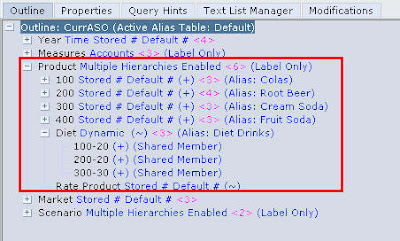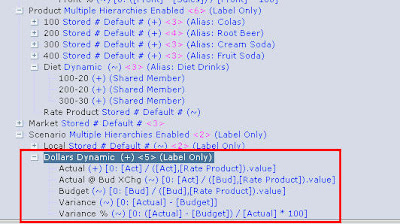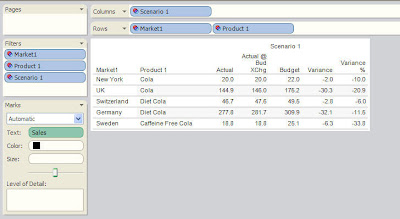Archive for December 31st, 2008

Hyperion Essbase 9.3.1 – Currency Conversion in ASO Cubes – Using MDX and Dynamic Hierarchies – Approach 1

Posted by Venkatakrishnan J on December 31, 2008

In the couple of blog entries here and here, we had seen an alternative approach to currency conversion in BSO cubes. One of the main reasons why i introduced that approach was to make sure that approach to currency conversion remains seamless as we move to ASO. The idea and the approach remains the same i.e we would be loading the rates and data within the same cube. The major difference between the currency conversion in ASO and BSO is that ASO currency conversion is dynamic i.e the conversion happens during data retrieval. In BSO the currency conversion happens during the data aggregation/calculation. To start with let us use the Aggregate Outline Migration Wizard to migrate the outline from BSO to ASO. When you migrate, ensure that you are using interactive migration so that you can modify the violating members manually. Following are the changes that one would have to make in the outline

1. Convert the Products dimension to have Multiple Hierarchies. And change the Diet member to be having a dynamic Hierarchy. This is because ASO does not support multiple shared members within a stored hierarchy.2. Convert the Scenario dimension to have multiple hierarchies. The idea is to load all the input Local currency data into the Stored local hierarchy. And all the conversions would happen under the Dollar dynamic hierarchy. We need to convert Dollar to dynamic hierarchy since ASO does not support calculations on non-Accounts dimensions.3. Change the input data for Rates to be loaded against Act and Bud intersection members instead of Actual and Budget.4. Create a new ASO cube and copy the members from the wizard to the ASO outline.

5. Copy the rule files that we created the other day from the BSO cube to the new ASO cube.

6. The outline conversion wizard does not convert the BSO formula automatically to ASO MDX formulas. So, change the formulas for the below shown members

```Margin % ----- ([Margin] - [Sales])/[Margin] * 100
Profit % ----- ([Profit] - [Sales]) / [Profit] * 100
Variance ----- [Actual] - [Budget]
Variance % ----- ([Actual] - [Budget]) / [Actual] * 100
```

7. This will ensure that the outline is now ASO compliant. In order to accomodate the currency conversion, we need to add formulas to the Actual, Budget and Actual @ Budget XChg members. The formulas are given below

```Actual ----- [Act] / ([Act],[Rate Product]).value
Budget ----- [Bud] / ([Bud],[Rate Product]).value
Actual @Budget XChg ----- [Act] / ([Bud],[Rate Product]).value
```As you see, all we are doing is we are making the Actual, Budget members to be dynamically calculated using the MDX formulas above.

8. Let us load the data using the same rule files which we copied over from BSO. Once that is done, let us look at the data to see whether the calculations are done correctly.As you see, the numbers above match exactly with what was there in the BSO cube. But the drawback with ASO is that, certain BSO specific features like Expense Reporting etc are not supported. These would have to be manually handled using MDX and UDAs. Also, the above approach might not be suitable in all the cases whenever retrieval performance is very important. For such cases, let us look at another approach in a future blog entry.

Belated New Year Wishes everyone!!!